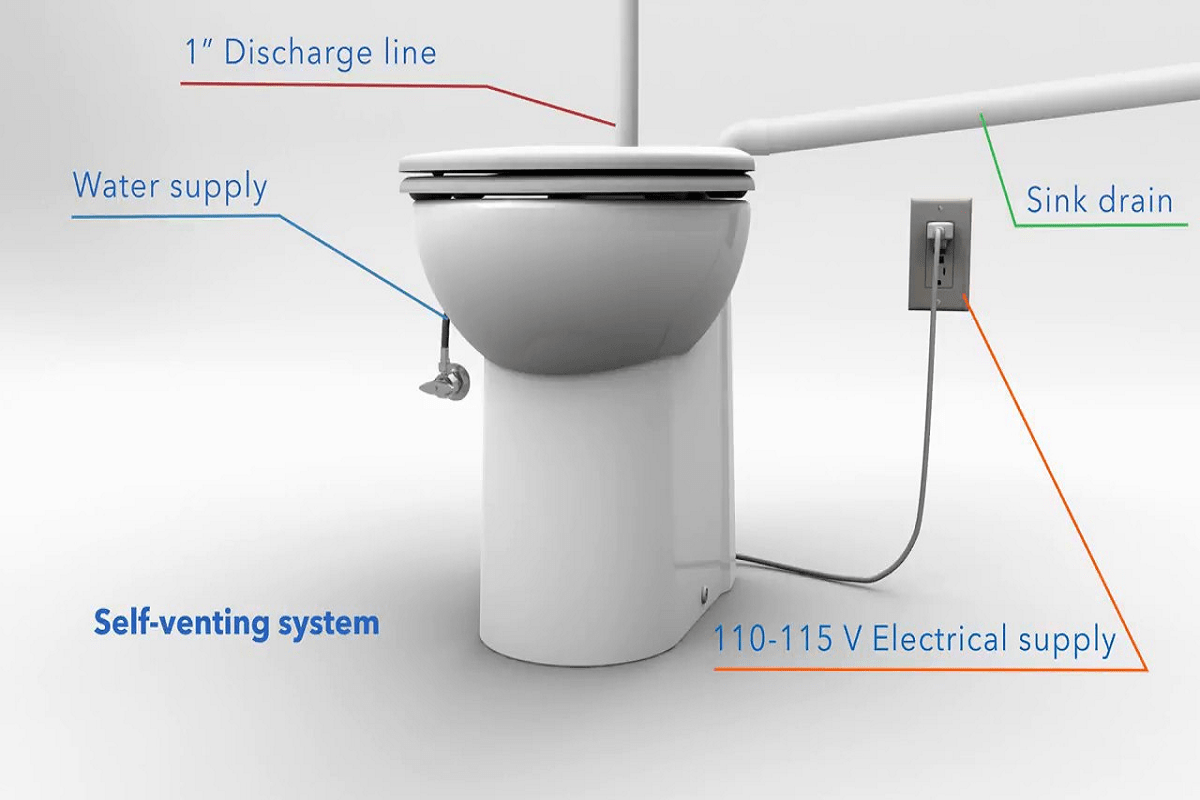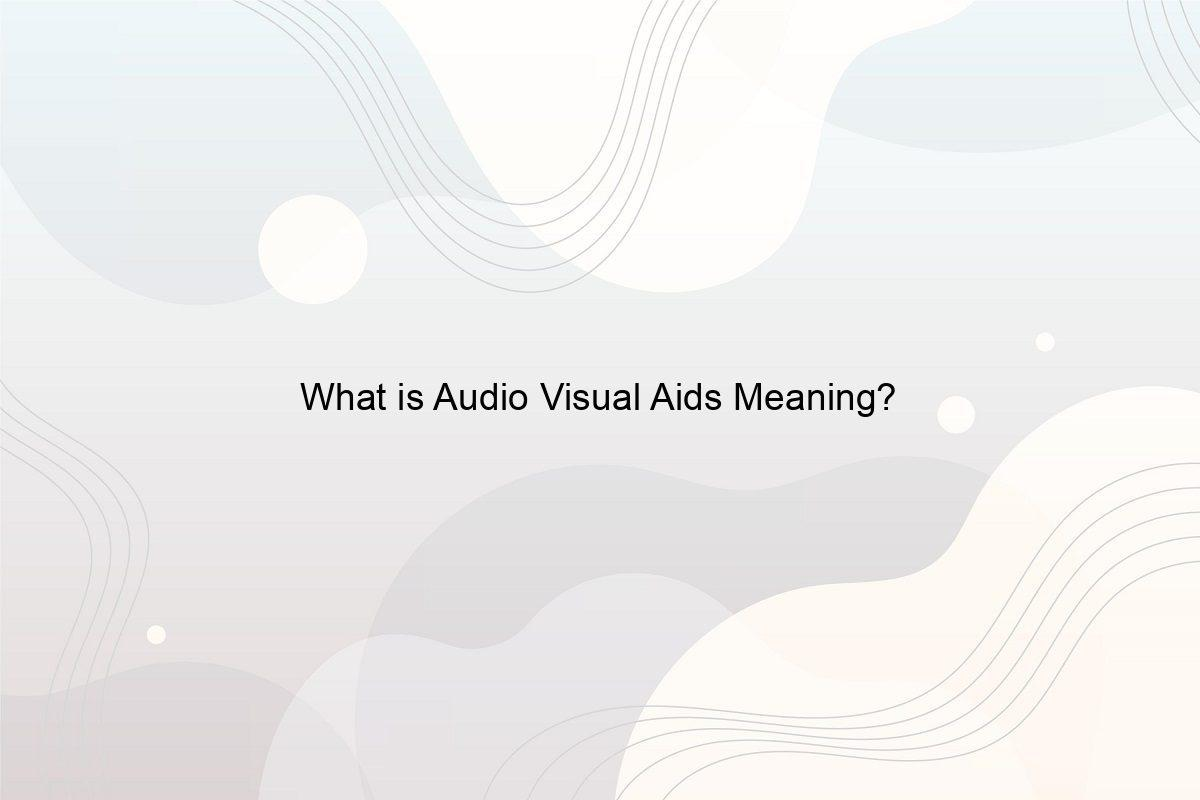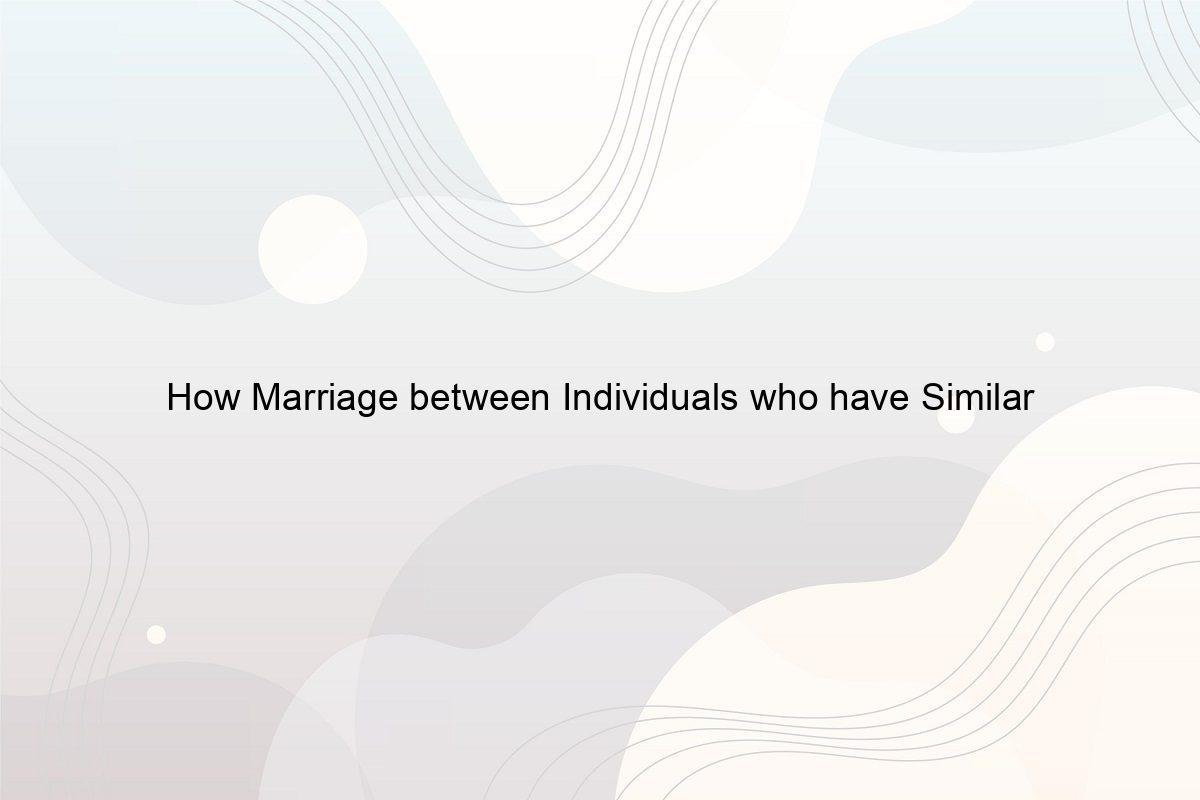﻿ What is Difference between Discovery Science and Hypothesis Driven Science? - Speeli

# What is Difference between Discovery Science and Hypothesis Driven Science?

What is Hypothesis-Driven Science? What is a Hypothesis-Driven Research? What is Discovery Science Primarily based on? What is the Relationship between the Scientific Method and Discovery? How do Hypotheses differ from Theories?

Science is so fascinating, isn’t it? But do you know there are different types of science? The two types which are considered important are discovery-based science and hypothesis-driven science. One is based on empirical and experimental research and the other is based on a tentative explanation. This article is going to look at the two types and what is the difference between discovery science and hypothesis driven science. We will also see the relationship between the scientific method and discovery and the multiple hypothesis testing in data science. Doesn’t it sound interesting? So let’s look at them!

### 1. What is Hypothesis-Driven Science?

Everything around us is a part of science, it may be the trees or even the pens with which you write. Science answers them all! Before understanding the difference between discovery science and hypothesis driven science, let us first understand the meaning of hypothesis-driven science. Hypothesis-driven science is a form of science that starts with a specific scientific explanation which is then tested.

Most scientific research is hypothesis-driven as it seeks to address a question that is specific, answerable, and measurable. If a hypothesis is well constructed, it will be clear, falsifiable, and testable. This science involves the usage of scientific methods for assessing explanations related to a phenomenon which is generally a published or a prior researched work. (See What are Properties in Science?)

### 2. What is a Hypothesis-Driven Research?

Hypothesis-driven research is when research is conducted based on a hypothesis developed. The hypothesis is a potential answer to the observation that was made. The research uses scientific tools to reach the results and form a conclusion. It tries to answer all the aspects of the observation that is being studied and is based on some tentative answers that are formed for the observation.

It tries to explain whether the hypothesis is true or not and the conclusion is made according to the result of the experiment. If the result does not support the hypothesis, then a new hypothesis has to be made and tested until the desired and appropriate result is achieved. (See Why is quantitative research important?)

### 3. What is Discovery Science Primarily based on?

By author Julia Koblitz on Unsplash

Discovery science is also known as discovery-based science. Discovery science is primarily based on the discovery of things or knowledge. It is about the prediction of outcomes in a certain situation. This science is mainly based on deductive reasoning, unlike hypothesis-driven science which is based on inductive reasoning. It mostly observes and describes nature. It mainly aims to observe and discover new things and knowledge in nature by using observational studies and detailed descriptions of events. (Also read How is Drawing used to Analyze the Movement of People or Material?)

### 4. What is the Relationship between the Scientific Method and Discovery?

Now that we know the meaning of discovery-based science, let us understand the relationship between the scientific method and discovery. The discovery of new things and knowledge leads to new observations and a better understanding of nature. The novel discovery-based research leads to the formulation of new hypotheses. Scientific methods are used to study these things through testing and experiments. Discovery is the finding of new things while the scientific method is the careful, detailed, and systematic study of a phenomenon which also means that discovery is an expansion of the scientific method. (See What is the Purpose of Database System?)

### 5. How do Hypothesis differ from Theories?

A hypothesis is an assumption made about a certain thing or phenomenon that has not been proven yet. It is made before any research so that the research can be conducted on it. A hypothesis is generally a starting point of any research whereas, a theory is a principle that is formed on data already existing. It is formed to explain things that already exist, directly and easily. A scientific theory incorporates laws, hypotheses, and facts. Check out How is Understanding Abstract Ideas done?

### 6. What is Difference between Discovery Science and Hypothesis Driven Science?

Photo by Scott Graham on Unsplash

Here, we are going to answer the difference between discovery science and hypothesis driven science.

• Discovery science is the scientific field that observes and discovers nature whereas hypothesis-driven science tries to explain nature. It discovers new knowledge and things. Discovery-based science focuses on studying more about discovered and found in nature.
• Deductive reasoning is used for discovery science whereas hypothesis-driven science is based on inductive reasoning. This is the major difference between discovery science and hypothesis driven science.

Hypothesis-driven science is a process that involves tentative answers to specific questions. The hypothesis begins with a specific problem which is tested to obtain a potential answer or solution to it. It is based on scientific theories that are already established. (See Why is Observing Patterns Important in Everyday Life?)

### 7. What is the Difference between Hypothesis Driven and Discovery Based Research?

Research that is based on scientific theories is hypothesis-driven research. This research is conducted after the theories that are scientific and can be studied deeply. In hypothesis-driven research, there is a hypothesis that might be used to explain a certain phenomenon. For hypotheses, doing prior research is necessary.

The discovery-based research does not call for any prior research. It is based on observational research. It does not have anything that needs to be proven like in hypothesis-driven research. Discovery-based research fully focuses on discovering new things in nature and then studying about them. (See What is a scientific question example?)

### 8. What is the Difference between Discovery Based Science and Hypothesis Testing?

After understanding the difference between discovery science and hypothesis driven science, let us also look at the difference between discovery based science and hypothesis testing.

In discovery-based science, the research is done by discovering new things and knowledge in the natural world. It aims to look out for things that are new and haven’t been found yet which can be studied from the start. Hypothesis testing is done on scientific theories.

In hypothesis testing, the research is based on some problem or findings that are tested to know if they are true or not. The hypothesis-driven testing is done with the aim of finding specific answers to a certain problem whereas discovery-based science focuses only on the discovery of new things in nature. (See What Graph is Useful for Showing Changes in a Variable?)

### 9. What is Multiple Hypothesis Testing in Data Science?

Hypothesis testing is a common scientific tool that is used in science and the data is then used to support the certainty of findings that are being studied through the hypothesis. Multiple hypothesis testing in data science is an act through which a number of individual hypothesis tests are considered simultaneously. The development of multiple hypotheses is made prior to the research and the same is done through the Null hypothesis and Alternative Hypothesis. (See What is the correct order of steps in the scientific method?)

### 10. What are the Proper Components of the Scientific Method?

By author Ousa Chea on Unsplash

The proper components of the scientific method are:

• Observation: An observation is made when something is noticed and is done after an experiment or research is conducted.
• Question: The question is framed after an observation is done and is based on the observation about how or why the thing is like that.
• Hypothesis: A hypothesis is a potential answer to the question we have framed and the answer that is proposed through the hypothesis is then tested to know if it is true or not. It is just a potential explanation to the question and is not always true.
• Prediction: The prediction is the outcome or result of the hypothesis that is proposed.
• Experiment: The experiment is the test for the hypothesis and is performed to see if the hypothesis that we have developed so far, is true or not. It has to be associated with the prediction that is made and then has to be tested accordingly.
• Results: Results are made on the basis of the experiment that was conducted. If the hypothesis has proven to be correct, then the results will reflect that and if it is incorrect, the results will be made accordingly. In the second case, a new hypothesis has to be developed which will be tested again.

Through this article, we are well aware of the difference between discovery science and hypothesis driven science. This article has covered in depth the different topics related to hypothesis-driven science and discovery-based science. Wasn’t it interesting to know about the relationship between the scientific method and discovery? I am sure you enjoyed this piece of writing which is based on the difference between discovery science and hypothesis driven science and some similar facts. So, come back for more! (Also read What is the Difference between Qualitative and Quantitative Observations?)

##### Related Posts## What Country am I in?

What is a Country? How many Countries are there in the World? What Country am I in if I live in Washington?## Can You Wet Vent a Saniflo System?

A saniflo toilet, or a macerator toilet, is different from a conventional toilet in its unique dual mode of operation. For further knowledge on the wet vent’s functionality, slide into the article.